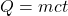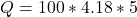## How much heat is required to raise 100 grams of water (c= 4.18) by 5 degrees Celsius?

Question

How much heat is required to raise 100 grams of water (c= 4.18) by 5 degrees Celsius?

in progress 0
5 months 2021-09-05T11:23:58+00:00 1 Answers 0 views 0

Heat capacity, Q = 2090 Joules.

Explanation:

Given the following data;

Mass = 100 grams

Specific heat capacity = 4.18 J/g°C.

Temperature = 5°C

To find the quantity of heat required;

Heat capacity is given by the formula;Where;

Q represents the heat capacity or quantity of heat.

m represents the mass of an object.

c represents the specific heat capacity of water.

t represents the temperature of an object.

Substituting into the formula, we have;Heat capacity, Q = 2090 Joules.